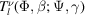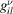International Tables for Crystallography (2019). Vol. H, ch. 5.2, pp. 538-554https://doi.org/10.1107/97809553602060000967

## Contents

• 5.2. Stress and strain  (pp. 538-554)
• 5.2.1. The importance of determining stress and the diffraction method  (p. 538) | html | pdf |
• 5.2.2. Strain and stress in single crystals, elastic constants and transformations  (p. 538) | html | pdf |
• 5.2.3. Strain and stress in polycrystalline samples  (pp. 540-542) | html | pdf |
• 5.2.3.1. Types of strains and stresses, and the strain/stress orientation distribution function  (pp. 540-541) | html | pdf |
• 5.2.3.2. The mean and variance of the observable strain, the peak shift and broadening  (pp. 541-542) | html | pdf |
• 5.2.4. Determining the strain/stress by diffraction  (pp. 542-543) | html | pdf |
• 5.2.4.1. Determining the macrostrain/stress  (pp. 542-543) | html | pdf |
• 5.2.4.2. Determining the microstrain  (p. 543) | html | pdf |
• 5.2.5. Macrostrain/stress in isotropic samples: classical approximations  (pp. 543-546) | html | pdf |
• 5.2.5.1. The Voigt model  (p. 544) | html | pdf |
• 5.2.5.2. The Reuss model  (pp. 544-545) | html | pdf |
• 5.2.5.3. The Hill average  (p. 545) | html | pdf |
• 5.2.5.4. The Kroner model  (p. 545) | html | pdf |
• 5.2.5.5. The sin2Ψ' method  (pp. 545-546) | html | pdf |
• 5.2.5.6. Determining the single-crystal elastic constants  (p. 546) | html | pdf |
• 5.2.6. The hydrostatic state in isotropic polycrystals  (pp. 546-547) | html | pdf |
• 5.2.7. Calculating the macroscopic strain/stress using spherical harmonics  (pp. 547-552) | html | pdf |
• 5.2.7.1. Strain expansion in generalized spherical harmonics: the Popa and Balzar approach  (pp. 547-548) | html | pdf |
• 5.2.7.2. The selection rules for all Laue classes  (p. 548) | html | pdf |
• 5.2.7.3. Generalized spherical harmonics of real type, and the WSODF index  (pp. 548-549) | html | pdf |
• 5.2.7.4. Determining the macrostrain/stress state of the sample  (pp. 549-550) | html | pdf |
• 5.2.7.5. Simplified (short') harmonics representation of the peak shift, and the mixed' representation  (p. 550) | html | pdf |
• 5.2.7.6. Implementation in Rietveld codes  (pp. 550-551) | html | pdf |
• 5.2.7.7. An application: determining the averaged macroscopic strain and stress tensors in a rolled uranium plate from time-of-flight neutron diffraction data  (pp. 551-552) | html | pdf |
• 5.2.7.8. Limitations of the spherical-harmonics approach and possible further developments  (p. 552) | html | pdf |
• 5.2.8. The spherical-harmonics approach to strain broadening  (pp. 552-553) | html | pdf |
• 5.2.8.1. Ignoring the macrostrain variance  (p. 552) | html | pdf |
• 5.2.8.2. The double-dependent anisotropic strain breadth (DDASB)  (pp. 552-553) | html | pdf |
• 5.2.8.3. The classical' limit of the DDASB  (p. 553) | html | pdf |
• References | html | pdf |
• Tables
• Table 5.2.1. The matrix C for all Laue groups represented by specific constraints  (p. 540) | html | pdf |
• Table 5.2.2. Quadratic and quartic forms for symmetries higher than triclinic  (p. 545) | html | pdf |
• Table 5.2.3. Assignment of functionsto the coefficients(p. 549) | html | pdf |
• Table 5.2.4. The real generalized spherical harmonics  (p. 549) | html | pdf |
• Table 5.2.5. The monomials jμl(a1, a2, a3) for l = 2, 4, 6  (p. 550) | html | pdf |
• Table 5.2.6. The complete set of polynomials Jμl for l = 2, 4, 6 for all Laue groups  (p. 551) | html | pdf |